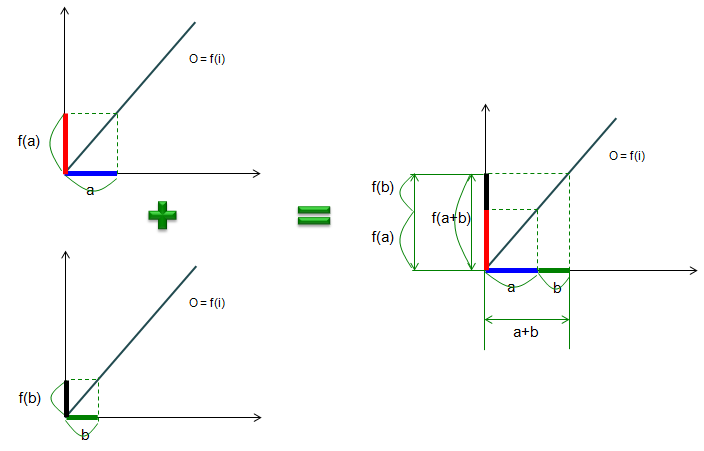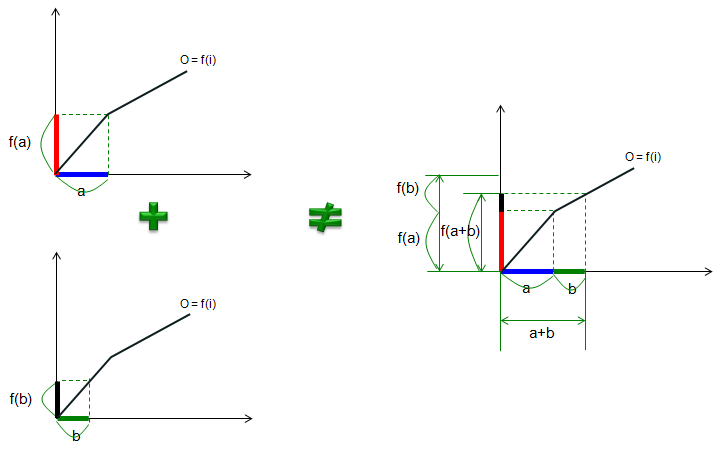Communication - Linearity                                 Home : www.sharetechnote.com One of the most important assumption for most of communication theory is that the system is LTI (Linear Time Invarient). So it is very important to understand the concept of LTI before you start getting deeper into communication technology. In this page, I will explain about what 'Linear' (Linearity) means and why it is important and in another page I will explain about 'Time Invarience'.   What does Linearity means ? you may think any function that can be expressed as a straight line in a coordinate system is linear. But it is not true. Not all straight line in the coordinate system is linear. For a function (system) to be a linear, it has to meet a criteria called 'principle of superposision'. In short, the principle of superposition is to make the following equation true.   f(a+b) = f(a) + f(b)   This principle can be explained in an illustration as shown below. Let's assum that you have a function f(x) as a straight line starting starting from the origin (0,0) of the coordinate system. Now look at the upper graph on left side first. If you plug a value 'a' (in thick blue line)' into the function. yoe will get the output of the function as f(a) (in thick red line). Now look at the bottom graph on left side first. If you plug a value 'b' (in thick green line)' into the function. yoe will get the output of the function as f(b) (in thick black line). Now let's take the graph of f(a+b). It is the graph on the right side. Just by looking, you would notice that f(a+b) is same as f(a) + f(b). Therefore, this graph(function, system) is linear.As an example, let's take we have a function as follows.   f(x) = 2 x   Is this a linear function ? Let's see if this meets the principle of superposition, i.e, f(a+b) = f(a) + f(b). Let's assume a = 3, b = 2.   Then what is f(a) ? It is f(3) = 2 * 3 = 6 what is f(b) ? It is f(2) = 2 * 2 = 4 Then what is f(a+b) ? It is f(2+3) = f(5) = 5 * 2 = 10. Is f(a+b) = f(a) + f(b) ? Yes, it is because f(3+2) = f(3) + f(2) = 6 + 4 = 10. Does this satisfy the principle of superposition ? (In strict mathematics, you cannot prove it with just single. You have to prove this is true for any numbers... try all different values as 'a' and 'b' and check if it still works -:). But intuitively, you can say "Yes, it satisfy the principle of superposition, so this function is a linear'.   If the explanation above is not clear to you, it would be clearer if you look at an example in which f(a+b) is not the same as f(a)+f(b). Following is an example. Look at the function graph in this case. It is not the straight line, it is a path kinked at one point (or you can draw a curved line as the function). Now look at the upper graph on left side first. If you plug a value 'a' (in thick blue line)' into the function. you will get the output of the function as f(a) (in thick red line). Now look at the bottom graph on left side first. If you plug a value 'b' (in thick green line)' into the function. yoe will get the output of the function as f(b) (in thick black line). Now let's take the graph of f(a+b). It is the graph on the right side. Just by looking, you would notice that f(a+b) is not same as f(a) + f(b). Therefore, this graph(function, system) is not linear.As an example, let's take we have a function as follows.   f(x) = x^2   Is this a linear function ? Let's see if this meets the principle of superposition, i.e, f(a+b) = f(a) + f(b). Let's assume a = 3, b = 2.   Then what is f(a) ? It is f(3) = 3^2 = 9 what is f(b) ? It is f(2) = 2^2 = 4 Then what is f(a+b) ? It is f(2+3) = f(5) = 5^2 = 25. Is f(a+b) = f(a) + f(b) ? No, it is because f(3+2) is 25 but f(3) + f(2) = 9 + 4 = 13. Does this satisfy the principle of superposition ? No. So it is not a linear   I hope you have the general concept of linearity by now. But I think practically more important question would be "Why the Linearity is so important ?", "Why we have to care about linearity ?".It is important because in linear system, once you have an output for any specific input, you can figure out all other outputs without doing real test.   For example, let's assume that you have a linear system and you know the outout of the system when you put '1' as an input is '2', i.e f(1) = 2 and somebody ask you to get the output when the input is 5, meaning that he wants to get 'f(5)'. In this case, by the principle of superposition you can rewrite the f(5) as follows.   f(5) = f(1) + f(1) + f(1) + f(1) + f(1)   Since you already know the value of f(1) = 2, you can get f(5) as follows without doing any test.   f(5) = f(1) + f(1) + f(1) + f(1) + f(1) = 2 + 2 + 2 + 2+ 2 = 10   You would see later that we usually indentify the characteristics of any linear system by what we call 'impulse response'. Once you have the impulse response of the system, you can figure out the output of the system for any input by using the impulse response. This is possible only when the system is Linear.